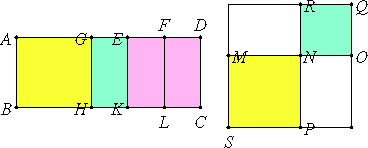# Proposition 58

If an area is contained by a rational straight line and the fifth binomial, then the side of the area is the irrational straight line called the side of a rational plus a medial area.

Let the area AC be contained by the rational straight line AB and the fifth binomial AD divided into its terms at E, so that AE is the greater term.

I say that the side of the area AC is the irrational straight line called the side of a rational plus a medial area.

Make the same construction shown before. It is then manifest that MO is the side of the area AC. It is then to be proved that MO is the side of a rational plus a medial area.Since AG is incommensurable with GE, therefore AH is also commensurable with HE, that is, the square on MN with the square on NO. Therefore MN and NO are incommensurable in square.

X.Def.II.5

Since AD is a fifth binomial straight line, and ED the lesser segment, therefore ED is commensurable in length with AB.

But AE is incommensurable with ED, therefore AB is also incommensurable in length with AE. Therefore AK, that is, the sum of the squares on MN and NO, is medial.

X.12

Since DE is commensurable in length with AB, that is, with EK, while DE is commensurable with EF, therefore EF is also commensurable with EK.

X.19

And EK is rational, therefore EL, that is, MR, that is, the rectangle MN by NO, is also rational.

Therefore MN and NO are straight lines incommensurable in square which make the sum of the squares on them medial, but the rectangle contained by them rational.

X.40

Therefore MO is the side of a rational plus a medial area and is the side of the area AC.

Therefore, if an area is contained by a rational straight line and the fifth binomial, then the side of the area is the irrational straight line called the side of a rational plus a medial area.

Q.E.D.

## Guide

This proposition is used in X.71.